Open in App
Not now

# PySpark create new column with mapping from a dict

• Last Updated : 23 Jan, 2023

In this article, we are going to learn about how to create a new column with mapping from a dictionary using Pyspark in Python.

The way to store data values in key: value pairs are known as dictionary in Python. There occurs a few instances in Pyspark where we have got data in the form of a dictionary and we need to create new columns from that dictionary. This can be achieved using two ways in Pyspark, i.e., using UDF and using maps. In this article, we will study both ways to achieve it.

### Methods to create a new column with mapping from a dictionary in the Pyspark data frame:

• Using UDF() function
• Using map() function

### Method 1: Using UDF() function

The most useful feature of Spark SQL & DataFrame that is used to extend the PySpark build-in capabilities is known as UDF, i.e., User Defined Function. In this method, we will see how we can create a new column with mapping from a dict using UDF. What we will do is create a function by using the UDF and call that function whenever we have to create a new column with mapping from a dictionary.

Stepwise Implementation:

Step 1: First of all, we need to import the required libraries, i.e., SparkSession, StringType, and UDF. The SparkSession library is used to create the session, while StringType is used to represent String values. Also, the UDF is used to create a reusable function in Pyspark.

```from pyspark.sql import SparkSession
from pyspark.sql.types import StringType
from pyspark.sql.functions import udf```

Step 2: Now, we create a spark session using getOrCreate() function.

`spark_session = SparkSession.builder.getOrCreate()`

Step 3: Then, we create a spark context.

`sc=spark_session.sparkContext`

Step 3: Later on, create a function to do mapping of a data frame to the dictionary which returns the UDF of each column of the dictionary.

```def translate(dictionary):
return udf(lambda col: dictionary.get(col), StringType())```

Step 4: Moreover, create a data frame whose mapping has to be done and a dictionary from where mapping has to be done.

```df = sc.parallelize([('column_value1', ),
('column_value2', ),
('column_value3', )]).toDF(['column_name'])```

Step 5: Further, create a data frame whose mapping has to be done and a dictionary from where mapping has to be done.

`dictionary = {'item_1': 'value_1', 'item_2': 'value_2', 'item_3': 'value_3', 'item_4': 'value_4'}`

Step 6: Finally, create a new column by calling the function created to map from a dictionary and display the data frame.

`df.withColumn("new_column", translate(dictionary_name)("column_for_mapping")).show()`

Example:

In this example, we have created a data frame with one column ‘key‘ from which new columns have to be created as follows: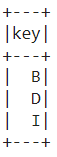Then, we created a dictionary from where mapping has to be done. Then, we created a new column ‘value‘ by calling the function which returns the UDF of each column of the dictionary created.

## Python3

 `# PySpark create new column with  ` `# mapping from a dict using UDF ` ` `  `# Import the libraries SparkSession, StringType and UDF ` `from` `pyspark.sql ``import` `SparkSession ` `from` `pyspark.sql.types ``import` `StringType ` `from` `pyspark.sql.functions ``import` `udf ` ` `  `# Create a spark session using getOrCreate() function ` `spark_session ``=` `SparkSession.builder.getOrCreate() ` ` `  `# Create a spark context ` `sc``=``spark_session.sparkContext ` ` `  `# Create a function to be called with mapping from a dict ` `def` `translate(dictionary): ` `    ``return` `udf(``lambda` `col: dictionary.get(col), ` `               ``StringType()) ` ` `  `# Create a data frame whose mapping has to be done ` `df ``=` `sc.parallelize([(``'B'``, ), (``'D'``, ), ` `                     ``(``'I'``, )]).toDF([``'key'``]) ` ` `  `# Create a dictionary from where mapping needs to be done ` `dictionary ``=` `{``'A'``: ``'Apple'``, ``'B'``: ``'Ball'``, ` `              ``'C'``: ``'Cat'``, ``'D'``: ``'Dog'``, ``'E'``: ``'Elephant'``} ` ` `  `# Create a new column by calling the function to map the values ` `df.withColumn(``"value"``, ` `              ``translate(dictionary)(``"key"``)).show()`

Output: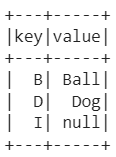Example 2: Creating multiple columns from a nested dictionary. In this example, we have created a data frame with column ‘Roll_Number‘ from which new columns have to be created as follows: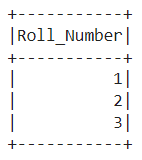Then, we created a dictionary from where mapping has to be done. Then, we created new columns ‘Name‘, ‘Subject‘, and ‘Fees‘ by calling the function which returns the UDF of each column of the dictionary created.

## Python3

 `# PySpark create new columns with  ` `# mapping from a dict using UDF ` ` `  `# Import the libraries SparkSession, StringType and UDF ` `from` `pyspark.sql ``import` `SparkSession ` `from` `pyspark.sql.types ``import` `StringType ` `from` `pyspark.sql.functions ``import` `udf ` ` `  `# Create a spark session using getOrCreate() function ` `spark_session ``=` `SparkSession.builder.getOrCreate() ` ` `  `# Create a spark context ` `sc``=``spark_session.sparkContext ` ` `  `# Create a function to be called with mapping from a dict ` `def` `translate(dictionary): ` `    ``return` `udf(``lambda` `col: dictionary.get(col), ` `               ``StringType()) ` ` `  `# Create a data frame whose mapping has to be done ` `df ``=` `sc.parallelize([(``1``, ), (``2``, ), ` `                     ``(``3``, )]).toDF([``'Roll_Number'``]) ` ` `  `# Create a dictionary from where mapping needs to be done ` `dictionary``=``{``'names'``: {``1``: ``'Vinayak'``, ` `                      ``2``: ``'Ishita'``, ` `                      ``3``: ``'Arun'``}, ` `            ``'subject'``: {``1``: ``'Maths'``, ` `                        ``2``: ``'Chemistry'``, ` `                        ``3``:``'English'``}, ` `            ``'fees'``:{``1``:``10000``, ``2``: ``13000``, ``3``: ``15000``}} ` ` `  `# Create a new column 'Name' by calling the function to map the values ` `df``=``df.withColumn(``"Name"``, ` `                 ``translate(dictionary[``'names'``])(``"Roll_Number"``)) ` ` `  `# Create a new column 'Subject' by calling the function to map the values ` `df``=``df.withColumn(``"Subject"``, ` `                 ``translate(dictionary[``'subject'``])(``"Roll_Number"``)) ` ` `  `# Create a new column 'Fees' by calling the function to map the values ` `df``=``df.withColumn(``"Fees"``, ` `                 ``translate(dictionary[``'fees'``])(``"Roll_Number"``)) ` ` `  `# Display the data frame ` `df.show()`

Output: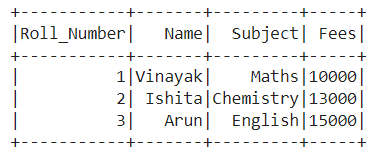### Method 2: Using map()

An RDD transformation that is used to apply the transformation function (lambda) on every element of RDD/DataFrame and returns a new RDD is known as map() function.  In this way, we will see how we can create a new column with mapping from a dictionary using the map. What we will do is convert each item of the dictionary to map type using the create_map() and call it to create a new column with mapping from a dictionary.

Stepwise Implementation:

Step 1: First of all, we need to import the required libraries, i.e., SparkSession, col, create_map, lit, and chain. The SparkSession library is used to create the session, while col is used to return a column based on the given column name. The create_map is used to convert selected DataFrame columns to MapType, while lit is used to add a new column to the DataFrame by assigning a literal or constant value. Also, the chain() function is used to link multiple functions.

```from pyspark.sql import SparkSession
from pyspark.sql.functions import col, create_map, lit
from itertools import chain```

Step 2: Now, we create a spark session using getOrCreate() function.

`spark_session = SparkSession.builder.getOrCreate()`

Step 3: Then, we create a spark context.

`sc=spark_session.sparkContext`

Step 4: Later on, create a function to do mapping of a data frame to the dictionary which converts each item of the dictionary to map type.

`mapping_expr = create_map([lit(x) for x in chain(*dictionary.items())])`

Step 5: Further, create a data frame whose mapping has to be done and a dictionary from where mapping has to be done.

```df = sc.parallelize([('column_value1', ),
('column_value2', ),
('column_value3', )]).toDF(['column_name'])```

Step 6: Finally, create a new column by calling the function created to map from a dictionary and display the data frame.

`df.withColumn("value", mapping_expr[col("key")]).show()`

Example:

In this example, we have created a data frame with one column ‘key‘ from which new columns have to be created as follows: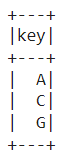Then, we created a dictionary from where mapping has to be done. Then, we created a new column ‘value’ by calling the create_map function which converts each item of the dictionary to map type.

## Python3

 `# PySpark create new column with  ` `# mapping from a dict using map ` ` `  `# Import the libraries SparkSession, col, create_map, lit, chain ` `from` `pyspark.sql ``import` `SparkSession ` `from` `pyspark.sql.functions ``import` `col, create_map, lit ` `from` `itertools ``import` `chain ` ` `  `# Create a spark session using getOrCreate() function ` `spark_session ``=` `SparkSession.builder.getOrCreate() ` ` `  `# Create a spark context ` `sc``=``spark_session.sparkContext ` ` `  `# Create a data frame whose mapping has to be done ` `df ``=` `sc.parallelize([(``'A'``, ), (``'C'``, ), ` `                     ``(``'G'``, )]).toDF([``'key'``]) ` ` `  `# Create a dictionary from where mapping needs to be done ` `dictionary ``=` `{``'A'``: ``'Apple'``, ``'B'``: ``'Ball'``, ` `              ``'C'``: ``'Cat'``, ``'D'``: ``'Dog'``, ` `              ``'E'``: ``'Elephant'``} ` ` `  `# Convert each item of dictionary to map type ` `mapping_expr ``=` `create_map([lit(x) ``for` `x ``in` `chain(``*``dictionary.items())]) ` ` `  `# Create a new column by calling the function to map the values ` `df.withColumn(``"value"``, ` `              ``mapping_expr[col(``"key"``)]).show()`

Output: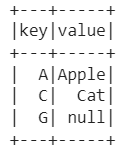My Personal Notes arrow_drop_up
Related Articles